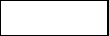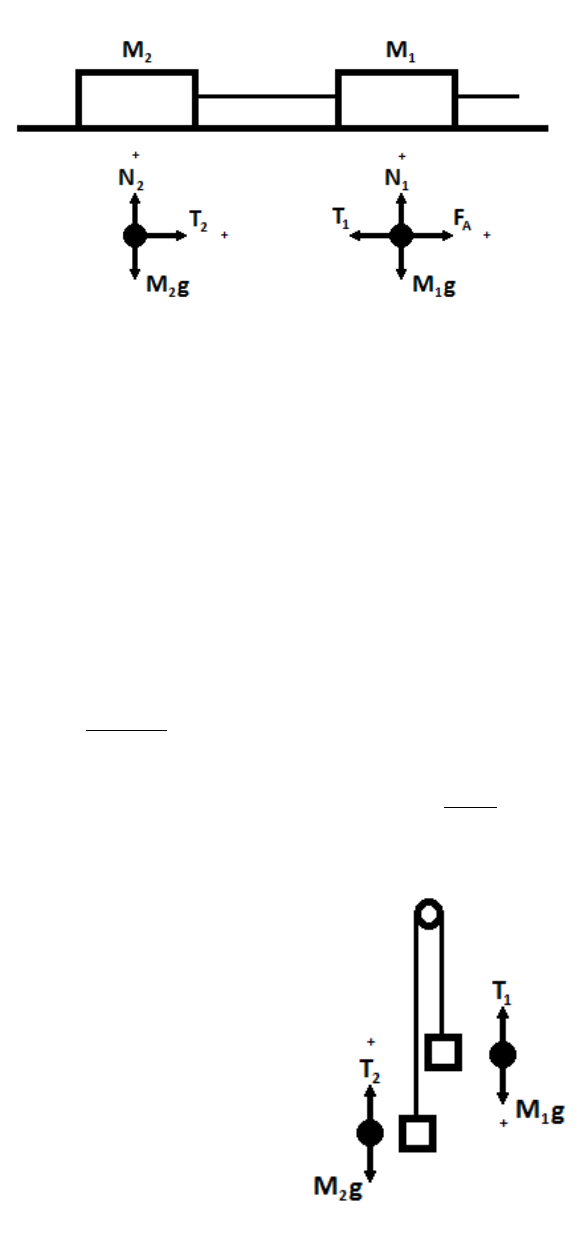# PHYS 2108 Lecture Notes - Lecture 4: Free Body Diagram, Net Force, Side Effect

295 views7 pages
School
Department
Course
Professor4-1
LAB 4:
Newtons Laws
In the vectors lab we allowed forces to interact with one another while they were balanced. This week,
we will allow unbalanced forces to show us what forces do.
Sir Isaac Newton put forth three laws of motion:
1. An object at rest stays at rest and an object in motion stays in motion at constant velocity;
unless acted upon by an outside force.
2. The force on an object is directly proportional to its mass and acceleration; and the direction
of acceleration is the same as the force acting on the object.
3. When one object exerts a force on a second object, the second object exerts an equal and
opposite force on the first.
Perhaps the most important one for us is the Second Law because it describes a relationship we can turn
into a useful equation. The net force on an object will determine the objects net acceleration as:

Eqn. 4-1:
Newtons Second Law
A side-effect that helps us understand the First Law is that if F = 0, then there must be zero acceleration.
An object at rest OR moving with a constant (unaccelerated) velocity will have no change if there is no
force applied or acceleration experienced.
There are several flavors of forces, but no matter where they originate they all behave the same way.
Applied forces are simply applied to a system from an outside push or pull.
Tension forces are due to strings. The tension on two objects at both ends of a string is usually
the same as a result of the Third Law, except in very special circumstances.
Frictional forces are a result of rubbing between an object and a surface. Frictional forces
usually act against the direction of motion, but sometimes are in the same direction of motion.
In those cases its sometimes called traction.
Normal forces dont mean ordinary. Theyre Third Law forces from surfaces your object
presses against, like the force from the chair onto you keeping you from sinking to the floor.
Normal means perpendicular to the surface.
When we analyze a situation, it is helpful to draw a free body diagram. That means we model each
object and draw vectors representing all forces that act upon that object. By having the vectors
explicitly drawn, it is easy to break them into components and see what happens in the x-, y-, and
maybe z- directions.
find more resources at oneclass.com
find more resources at oneclass.com
Unlock document

This preview shows pages 1-2 of the document.
Unlock all 7 pages and 3 million more documents.4-2
Consider the following situation. Two blocks are connected by a string on a frictionless surface. Block 1
is being pulled by an applied force to the right. Find the acceleration of the blocks.
To solve force problems:
1. Draw Free Body Diagrams
2. Choose + Directions
3. Break into components
(if applicable)
4. Write equations
In x:
 
 
 
In y:
   
   
5. Make assumptions. In this case T1 = T2 because its the same string, and due to Newtons Third
Law. Also both accelerations are the same if the string is not allowed to stretch. The y-direction
solutions are trivial and unhelpful in finding the acceleration.
  , and   
6. Substitute and solve. Im subbing the tensions because I dont want them.
 
  
 
7. If we wanted the tension, its easily solvable now. Just plug this acceleration into either tension
equation. They both give you same result.
Pulleys are a different, but similar scenario. There are no normal
forces because they are not pressing against a surface, and up is
positive in one case but down in the other. This is because as one
block falls the other rises, and the direction is carefully chosen to